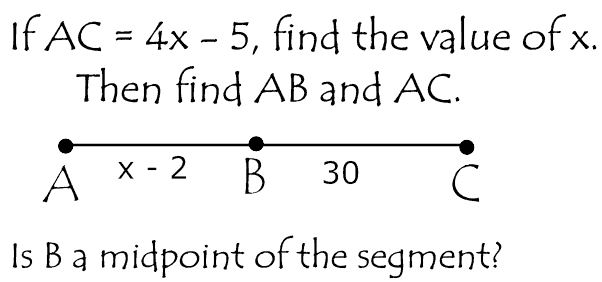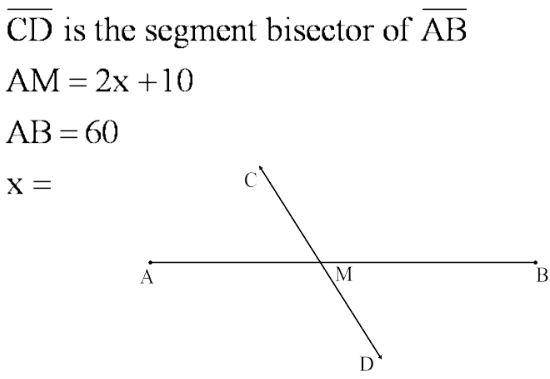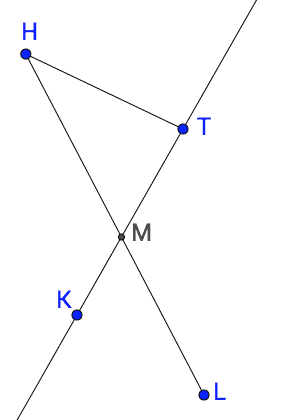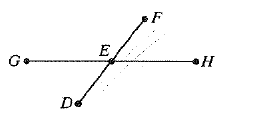1.1.2 Lines, Segments, Rays and Angles
starstarstarstarstarstarstarstarstarstar
by Jason Appel
| 25 Questions
Note from the author:
Playlist
By the end of the playlist, I can:
-name and identify 3 undefined terms in geometry (point, line, plane)
-explain the difference between congruent and equal
-name angles properly
-understand the difference between congruent and equal
1
1
I've watched the video or completed a mini-lesson on difference between congruent and equal explained.
True
False
2
1
I've watched the video or completed a mini-lesson on segment addition postulate explained with example.
True
False
3
1
I've watched the video or completed a mini-lesson on example using segment addition and midpoints.
True
False
4
1
I've tried these practice problems on the segment addition postulate and am confident that I know how to do them. copy and paste into a new tab: https://www.khanacademy.org/math/in-seventh-grade-math/simple-equations/new-topic-2015-11-13T01:43:29.211Z/e/segment_addition
True
False
5
1
I've tried these problems on midpoints and am confident that I know how to do them. copy and paste into a new tab: https://www.khanacademy.org/math/in-seventh-grade-math/simple-equations/new-topic-2015-11-13T01:43:29.211Z/e/midpoint_of_a_segment
True
False
6
1
I've watched the video or completed a mini-lesson on naming angles.
True
False
7
1
I've tried these practice problems on naming angles and am confident that I know how to do them. copy and paste into a new tab: https://www.khanacademy.org/math/basic-geo/basic-geo-angle/angle-intro/e/naming-angles
True
False
8
1
I've watched the video or completed a mini-lesson on complementary, supplementary, vertical angles, linear pair.
True
False
9
1
I've tried these problems on finding measures of complementary and supplementary angles and am confident I know how to do them. copy and paste into a new tab: https://www.khanacademy.org/math/basic-geo/basic-geo-angle/vert-comp-supp-angles/e/complementary_and_supplementary_angles
True
False
10
1
I've tried these problems on finding measures of vertical angles and am confident that I know how to do them. copy and paste into a new tab: https://www.khanacademy.org/math/basic-geo/basic-geo-angle/vert-comp-supp-angles/e/vertical_angles
True
False
11
1
I've watched the video or completed a mini-lesson on defining an angle bisector.
True
False12
13
14
15
16
12
10
x=?
13
10
AB = ?
14
10
AC = ?
15
10
Is B the midpoint of line segment AC?
Yes
No
Cannot be determined17
17
10
x=?
In the figure below, lines HL and KT intersect at the midpoint of segment HL.18
19
20
21
22
23
18
10
True or False: LM=MH
True
False
19
10
KM must equal MT.
True
False
20
10
Line MT bisects segment LH
True
False
21
10
Ray MT and ray TM are opposite rays.
Not sure what the term “opposite rays” means? Read this [http://www.mathopenref.com/oppositerays.html].
True
False
22
10
Ray MT and ray MK are opposite rays.
True
False
23
10
HM + ML = HL
True
False
E is the midpoint of segment DF.24
25
24
10
If DE = 5x + 3, and EF =33, then x = ?
25
10
If GE = y, EH = y-1, and GH = 11, then y = ?